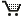# On Parametric Semi-infinite Optimization

Oliver Stein

Abstract. For one-parametric finite optimization problems it is well known that, in the generic case, each generalized critical point belongs to one of exactly five different types, where the singular points (types 2 to 5) form the boundary of the set of non-degenerate critical points (type 1). The local structure of the generalized critical point set at the singular points is well understood, and pathfollowing algorithms which are based upon these results have been implemented. The goal of this thesis is to give a corresponding type classification for generic one-parametric semi-infinite optimization problems, to study the local structure of the generalized critical point set at the singular points and to suggest an appropriate pathfollowing algorithm.

It turns out that in semi-infinite programming the five types from the finite case as well as three additional singular types of generalized critical points (in the sequel shortly: g.c.points) occur. We call these points to be of type 6, 7 and 8; in the latter case we distinguish points of type 8a and 8b. We review the finite type classification in detail because, firstly, we use the Lagrange multiplier notation of Poore/Tiahrt and need to restate known results in this notation and, secondly, we clarify the relationship between the finite type classifications of Hettich/Rupp and Jongen/Jonker/Twilt. Moreover, we give detailed genericity proofs for the finite case so that in the genericity proofs for the semi-infinite case we can concentrate on the peculiarities there.

At points of type 6 and 8a, the g.c.point set is the union of two branches which meet at the singular point under a non-vanishing angle (unless the total number of active indices equals the state space dimension at a point of type 6). According to its characteristic numbers, a point of type 6 can be a turning point of the g.c.point set, and we give formulas for the change of the quadratic indices. Furthermore, we suggest an explicit jump direction when the set of local minimizers ends at a turning point of type 6. A point of type 8a cannot be a turning point, and the quadratic index remains constant.

Points of type 7 and 8b are characterized by the violation of the Mangasarian-Fromovitz constraint qualification at the degenerate index in the lower level problem. In fact, the corresponding component of the index set vanishes locally under pertubations of the parameter. As a consequence, the feasible set mapping is not upper semi-continuous at points of type 7 and 8b, and these points are endpoints of the g.c.point set. However, we find explicit jump directions if the latter set consists of local minimizers.

By a related effect, a path of non-degenerate critical points can possess non-feasible endpoints, called trap-door points, so that the set of g.c.points is not necessarily closed in parametric semi-infinite programming, in contrast to the finite case. Under the additional assumption of lower semi-continuity for the index set mapping, neither trap-door points nor g.c.points of type 7 and 8b occur. Then the "typically semi-infinite" degeneracies reduce to points of type 6 and 8a, and the g.c.point set is closed.

Based on these results we suggest pathfollowing algorithms which trace the set of Fritz John points and the set of local minimizers, respectively.Order your copy at the publisher's web page.